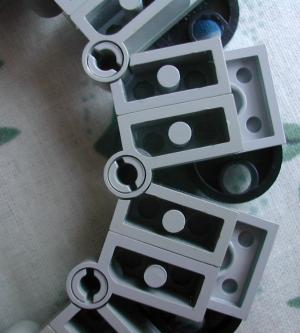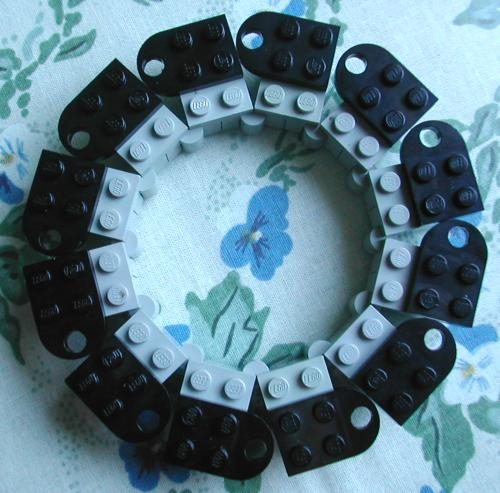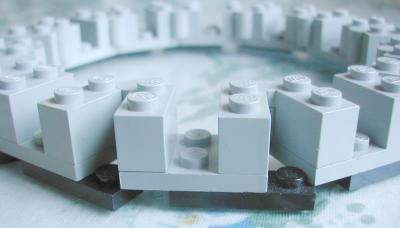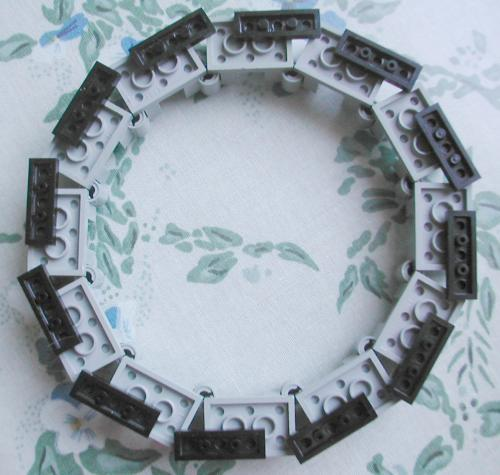# Saturn

For this ride I had to build a platform in the shape of a ring.
The diameter should be about 30 studs. To how many parts should I devide the ring?
The length of the ring is the diameter times PI ,that is 30 studs * 3.14159 = 94.248 studs
In the real life the platform parts are as long as the trailer is wide so it's 8 studs.
94.248 studs devided by 8 studs = 11.78 so it means 12 parts.

Next I had to know the angle between the parts. That's easy: The full circle is 360 degrees.
360 degrees / 12 = 30 degrees. This is a good angle because sin30 = 0.5 = 1/2
It means a triangle where one of the sides is 1, another is 2 and angle A is 30 degrees.This way I could build a ring that is round and that doesn't need any bars in the middleI made the structure even simpler: